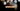# Leetcode | Solution of Majority Element in JavaScript

April 5th, 2020
|

In this post, we will solve Majority Element from leetcode and compute it's time and space complexities. Let's begin.

# Problem Statement

The question can be found at leetcode Majority Element problem.

The problem states that we are given an array and we need to find the majority element in the array. The majority element is the element that is present more than `n/2` times in the array, where `n` is the length of the array.

# Solution

Since the question mentions that majority element does exist in the array, we can sort the array and the middle element is the majority element. Since the number has to be more than `n/2` times, it has to be in the center. A solution would look something like this

``````var majorityElement = function (nums) {
nums.sort((a, b) => a - b);

const l = nums.length;

if (l % 2 === 0) {
return nums[l / 2]
} else {
return nums[(l - 1) / 2]
}
};``````

First, we sort the array, and next, we find the middle element. If the length is even, we find the center by dividing the length by 2 else we subtract 1 from length and then find the center.

This would be O(n2) in the worst case due to sorting, in terms of time complexity. Can we do better?

# Boyer–Moore majority vote algorithm

We will use Boyer–Moore majority vote algorithm to find the majority element in linear time complexity.

The algorithm works when there is a guarantee that the majority element exists. It is based on a very simple concept that the count of the majority will be greater than the combined count of all the other values in the array. Here's the algorithm

• First, we assume that element at index `0` is our majority element
• We declare two variables `maj` and `count` to keep the index of majority element and its count
• We will initialize these variables with value `0,1` respectively i.e the index at which majority element exists is `0` and its count is `1`
• Now we will iterate over the array from index `1`
• When we encounter an element, if it is same as the number at majority index, we increase the count else decrease it
• If at any point the count becomes `0`, we change majority index to that current index and change count back to `1`
• At last, we have our index where the majority element exists

If the majority index exists, it's count will be more than count of all the numbers combined. Hence we always get the index at which the majority index exists

We have discussed the approach, I urge you to go ahead on leetcode and give it another try.If you are here, it means something went wrong in implementation or you are just too lazy. In any case, let's see a simple implementation of the above logic.
``````var majorityElement = function (nums) {
let maj = 0, count = 1;

for (let i = 1; i < nums.length; i++) {
if (nums[i] === nums[maj]) {
count++
} else {
count--;
}

if (count === 0) {
maj = i;
count = 1;
}
}
return nums[maj]
};``````

The code here is the straight forward implementation of the algorithm. In case you still have doubts, please check the video below.

Here are the stats on submission

``````Status: Accepted
Runtime: 64ms
Memory: 37.5MB``````

## Time and space complexity

### Time complexity

We are looping over the array once, so time complexity would be O(n).

### Space complexity

We are using extra space to store a couple of variables. So space complexity would be constant, O(1).

# Summary

So, we solved the Majority Element problem by using Boyer-Moore Majority Voting Algorithm and calculated the time and space complexities.

I hope you enjoyed solving this question. This is it for this one, complete source code for this post can be found on my Github Repo. Will see you in the next one.